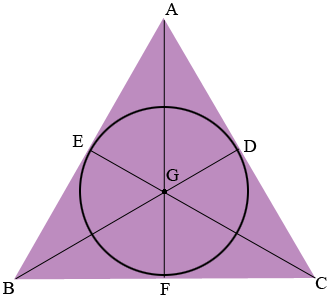SEARCH HOMEMath Central Quandaries & QueriesQuestion from jo, a student: what is the radius of the inscribed circle of an equilateral triangle with altitude 12 units?Hi Jo,In my diagram D, E and F are the points of tangency of the inscribed circle to the sides of the equilateral triangle. By the symmetry of the circle the lines AF, BD and CE pass through the center G of the circle . Can you use this diagram to show that the length of FG is one-third the length of FA?

PennyMath Central is supported by the University of Regina and the Imperial Oil Foundation.